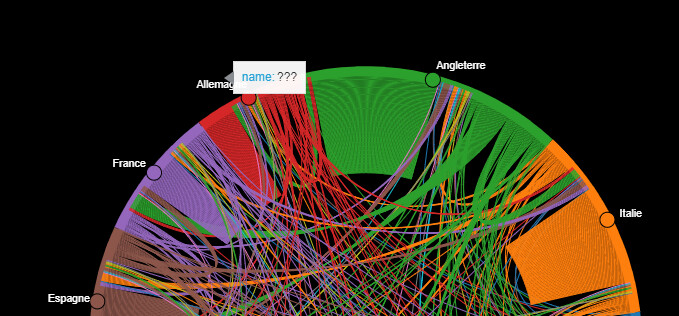# Get rid of "?" pop up - Holoviews Chord Diagram

Hello,
I’ve been facing a tiny issue with my python code when using HoloViews to make a chord diagram. It works perfectly fine, the only issue is when the graph is rendered, there’s this pop up that gets stuck whenever you hover over the label of the nodes…
Here’s an example:My code:

``````%%opts Chord [height=600 width=600 title="Transactions from 2000 to 2021" ]
#plot
#edit links = moves[moves['Transactions'] > x] // x refers to the number of transactions minimum for the diagram display
moves = moves[moves['Transactions']>5]
chord
nodesl = []
c = []
for i, row in moves.iterrows():
c.append(row)
c.append(row)

c = pd.DataFrame(c)
c.drop_duplicates(inplace=True)
c.reset_index(inplace=True)
c.drop(columns='index', inplace=True)
c

nodes = []
for i, row in c.iterrows():
nodes.append({'name':row})

nodes = pd.DataFrame(nodes)
# nodes

nodes = hv.Dataset(nodes, 'name')

%%opts Chord [height=800 width=800  bgcolor="black"]
%%opts Chord [title="Transactions from 2000 to 2021 (Countries with over 5 moves)\nTip: Please do not hover over the label as it might produce a bug, else refresh the page" ]

#this function allows text to fit perfectly on the screen
def rotate_label(plot, element):
text_cds = plot.handles['text_1_source']
length = len(text_cds.data['angle'])
text_cds.data['angle'] = *length
xs = text_cds.data['x']
text = np.array(text_cds.data['text'])
xs[xs<0] -= np.array([len(t)*0.019 for t in text[xs<0]])

chord.opts(
opts.Chord(cmap='Category10',
edge_color=dim('Target').str(),
node_color=dim('name').str(),
labels='name',
label_text_color="white",
hooks=[rotate_label]
))
chord

``````

Maybe you can disable the tooltips? Try setting `.opts(tools=[])` or something similar. Inspiration might be found in the section on tools and tooltips in Plotting with Bokeh — HoloViews 1.14.5 documentation.

It does work disabling the tools, however it won’t produce an interactive report to export it to an html file later on, which is what I want…

Could you be more specific? Why won’t that work?

It did work, except that when I disabled the tools, I can no long click on the nodes to visualize the links…

This is what I want to see with every individual node

1 Like